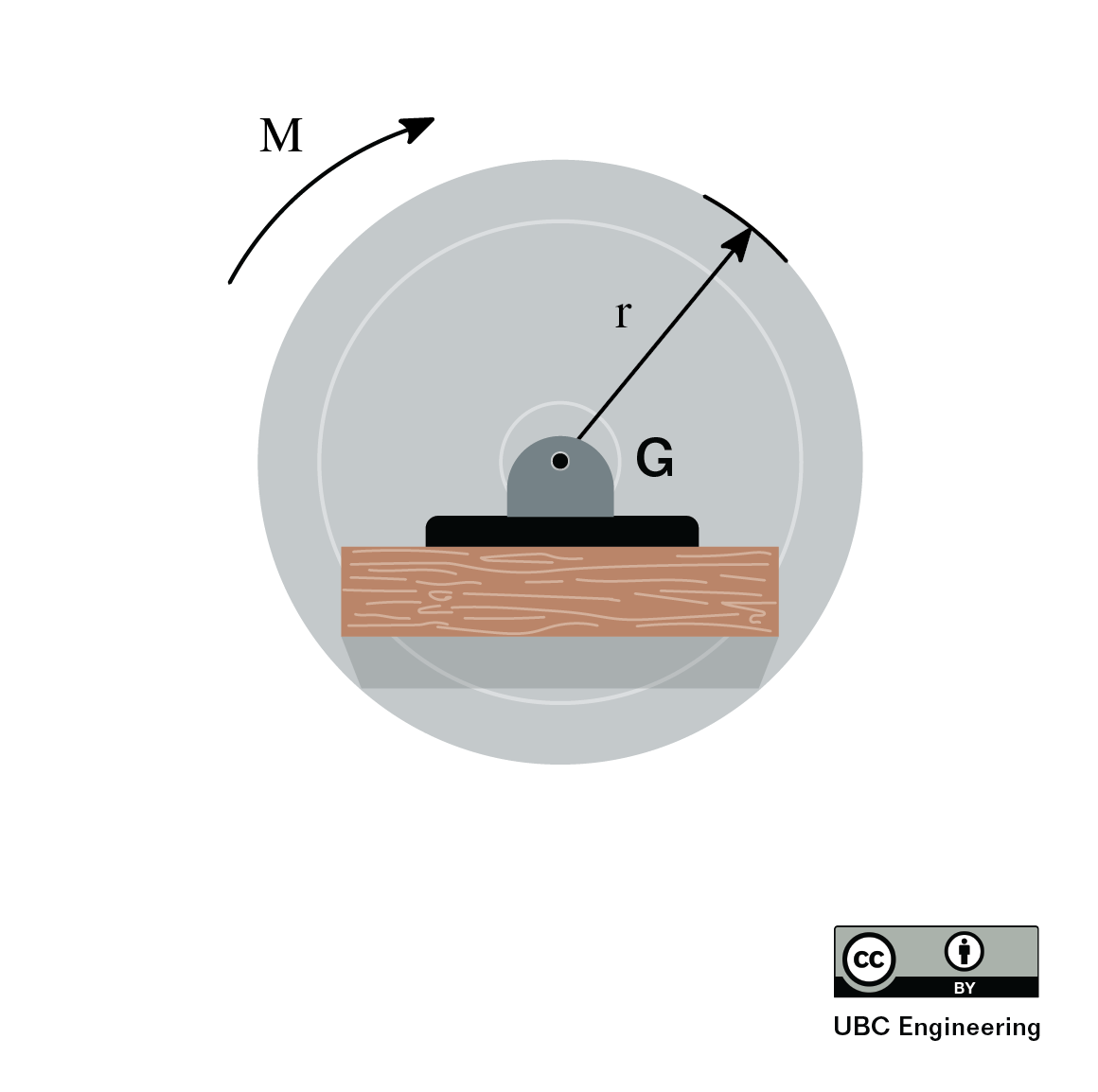﻿ Mechanics Map - Fixed Axis Rotation (Vector)

# Fixed Axis Rotation in Rigid Bodies Using Vectors

This section presents relative motion using vector notation.

When moving from particle kinematics to rigid body kinematics, we add the rotation of a body into the motion analysis process. Some bodies will translate and rotate at the same time, but many engineered systems have components that simply rotate about some fixed axis. We will start our examination of rigid body kinematics by examining these fixed axis rotation problems, where rotation is the only motion we need to worry about.The flywheel on this antique motor is a good example of fixed axis rotation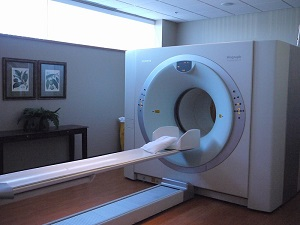The rotating x ray tube within the gantry of this CT machine is another example of fixed axis rotation. Image by Thirteen of Clubs CC-BY-SA 2.0

## Angular Position, Velocity, and Acceleration.

Just as with translational motion, we will have angular positions, which we can take the derivative of to find angular velocities, which we can again take the derivative of to find angular accelerations. Since we can only have a single axis of rotation in two dimensional problems (rotating about the z axis, with counter-clockwise rotations being positive, and clockwise rotations being negative) the equations will mirror the one dimensional equations used in particle kinematics.

Angular Position: $\theta(t)$ $\omega(t)=\frac{d\theta}{dt}=\dot{\theta}$ $\alpha(t)=\frac{d\omega}{dt}=\frac{d^2\theta}{dt^2}=\ddot{\theta}$

Also as with one dimensional translational motion, we can use integration to move in the opposite direction (just remember your constants of integration).

Acceleration: $\alpha(t)$ $\omega(t)=\int \alpha(t)$ $\theta(t)=\int \omega(t)=\iint \alpha(t)$

If we have constant angular accelerations, we can also use the following formulas adapted from one dimensional motion.

Acceleration: $\alpha(t)=\alpha$ $\omega(t)=\alpha t+\omega_{0}$ $\theta(t)=\frac{1}{2}\alpha t^{2}+\omega_{0}t+\theta_{0}$ $\omega^{2}-\omega{_{0}}^{2}=2\alpha(\theta-\theta_{0})$

## Velocity and Acceleration of a Point on a Rotating Body:

With fixed axis rotation, there is a single point on a body that does not move, however all other points on this body will have some velocity and some acceleration due to the rotation of the body itself.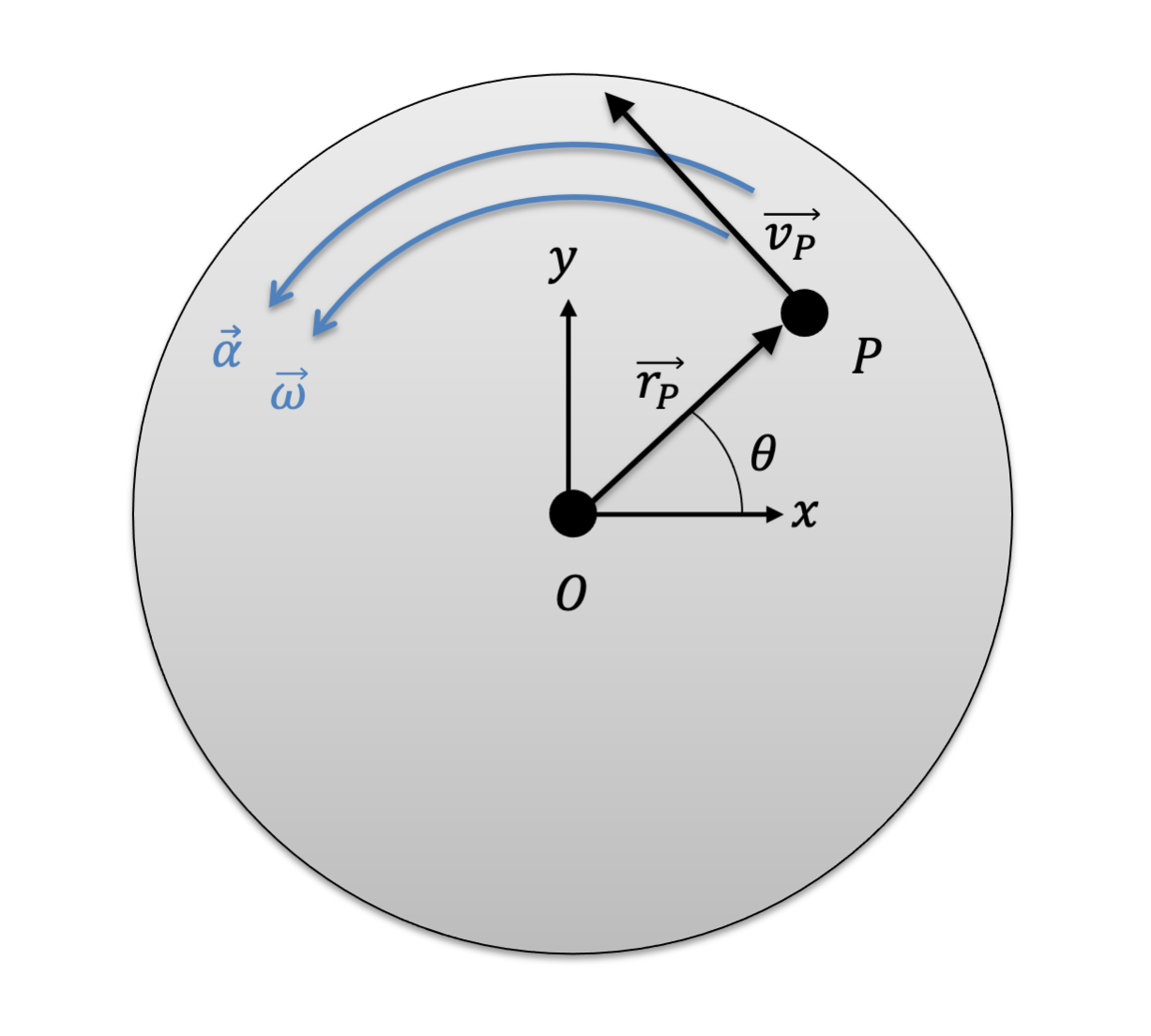The diagram above shows a point P on a body rotating about fixed axis O. Point P is a constant distance r away from the fixed point O. We will derive vector equations to find the velocity and acceleration of point P.

To determine the velocities and accelerations of these points, we will derive vector equations in rectangular coordinates. First, we decompose the position vector rP into x and y components.

Position of P with respect to the fixed axis: $\vec{r_P} = r_P cos\theta \hat{i} + r_P sin \theta \hat{j}$

We will then take the derivative of rP with respect to time, using the product rule. This will give us the velocity of P.

 $\vec{v_P} = \frac{d}{dt}(r_P) cos\theta \hat{i} + r_P \frac{d}{dt}(cos\theta) \hat{i} + \frac{d}{dt}(r_P) sin \theta \hat{j} + r_P \frac{d}{dt}(sin \theta) \hat{j}$

To simplify the above equations, we can note that for a rigid body, the point P never gets any closer or further away from the fixed center point O. This means that the distance rP never changes and the derivatives of rP in the above equations are zero. Putting this to work, we can simplify the above equation into the equation below.

 $\vec{v_P} = r_P \frac{d}{dt}(cos\theta) \hat{i} + r_P \frac{d}{dt}(sin \theta) \hat{j}$ $\vec{v_P} = r_P (-sin \theta) \dot{\theta} \hat{i} + r_P (cos\theta) \dot{\theta} \hat{j}$

Rearranging the equation, we can find the final expression we will use.

 $\vec{v_P} = \dot{\theta} \hat{k} \times (r_P cos\theta \hat{i} + r_P sin \theta \hat{j})$ $\mathbf{\vec{v_P} = \vec{\omega} \times \vec{r_P}}$

In a similar way, we can find an expression for the acceleration of point P.

 $\vec{a_P} = \vec{\alpha} \times \vec{r_P} + \vec{\omega} \times (\vec{\omega} \times \vec{r_P})$

For planar motion, the vectors omega and rP will always be perpendicular to each other, so we can simplify further.

 $\mathbf{\vec{a_P} = \vec{\alpha} \times \vec{r_P} - \omega^2 \vec{r_P}}$

The acceleration of point P has two components. Note that the omega-squared in the second term is not a vector - it's the scalar value of omega. The direction of that term is defined by the vector rP. In planar motion, the two components are perpendicular to each other. We can describe them as occurring in the normal and tangential directions.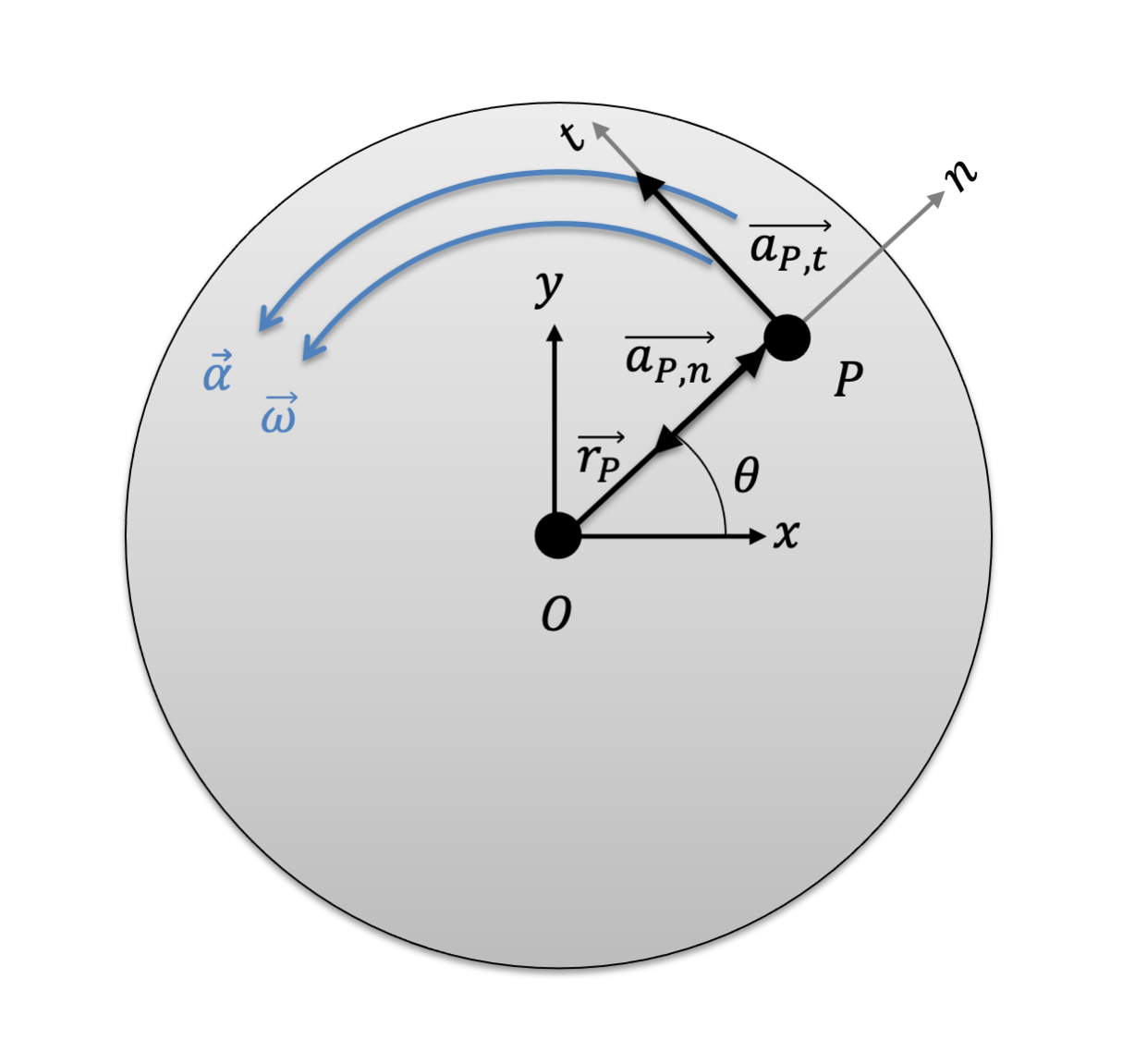The diagram above shows the acceleration components at point P on a body rotating about fixed axis O. The acceleration components can be described as occurring in the normal and tangential directions.
 $\vec{a}_{P,t} = \vec{\alpha} \times \vec{r}_{P}$ $\vec{a}_{P,n} = - \omega^2 \vec{r}_{P}$

These equations allow us to find the velocity and acceleration of any point on a body rotating about a fixed axis, given the vectors for angular velocity of the body (omega), the angular acceleration of the body (alpha), and the position of the point with respect to the axis of rotation (rP, or alternately written as rP/O).

## Worked Problems:

### Question 1:

A flywheel rotates on a fixed axle in a steam engine. The flywheel is rotating at a rate of 600 rpm before a brake begins decelerating the flywheel at a constant rate of 30 rad/s2. What is the time required to bring the flywheel to a complete stop? How many rotations does the flywheel go through while decelerating?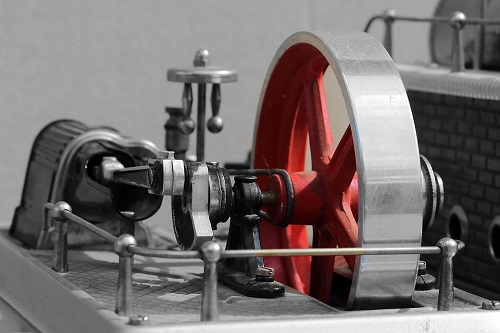### Question 2:

An 8 cm diameter hard drive platter is rotating at a constant rate of 3600 rpm counterclockwise. What is the velocity of a point, P, on the outer edge of the platter? What is the acceleration experienced by a point, P, on the edge of the platter?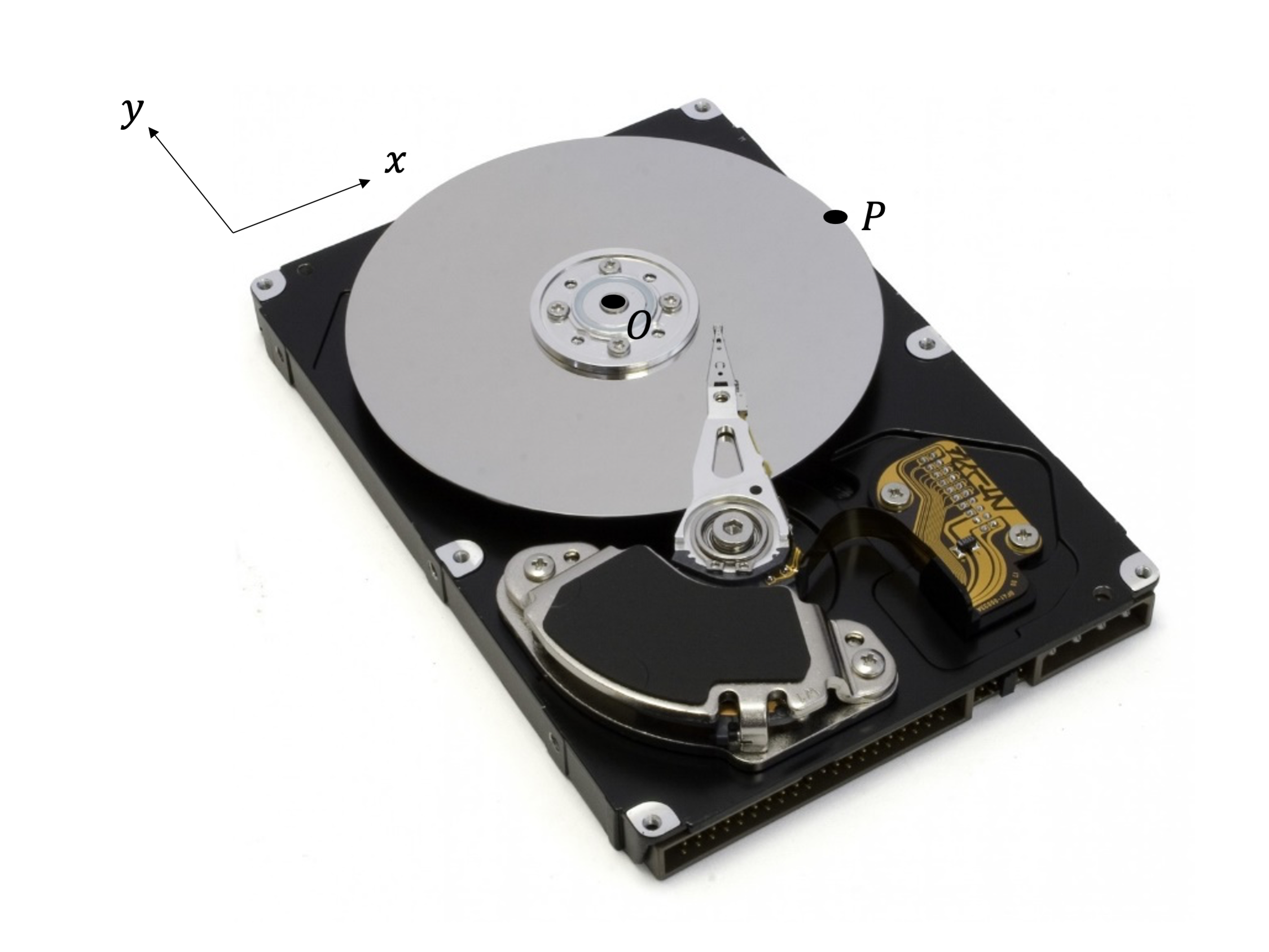### Question 3:

An 8 cm diameter hard drive platter is rotating at a constant rate of 3600 rpm counterclockwise. What are the velocity and acceleration of a point, P, under the read/write head? The head is 1.5 cm from the platter axis, and located at an angle of 45 degrees from the horizontal.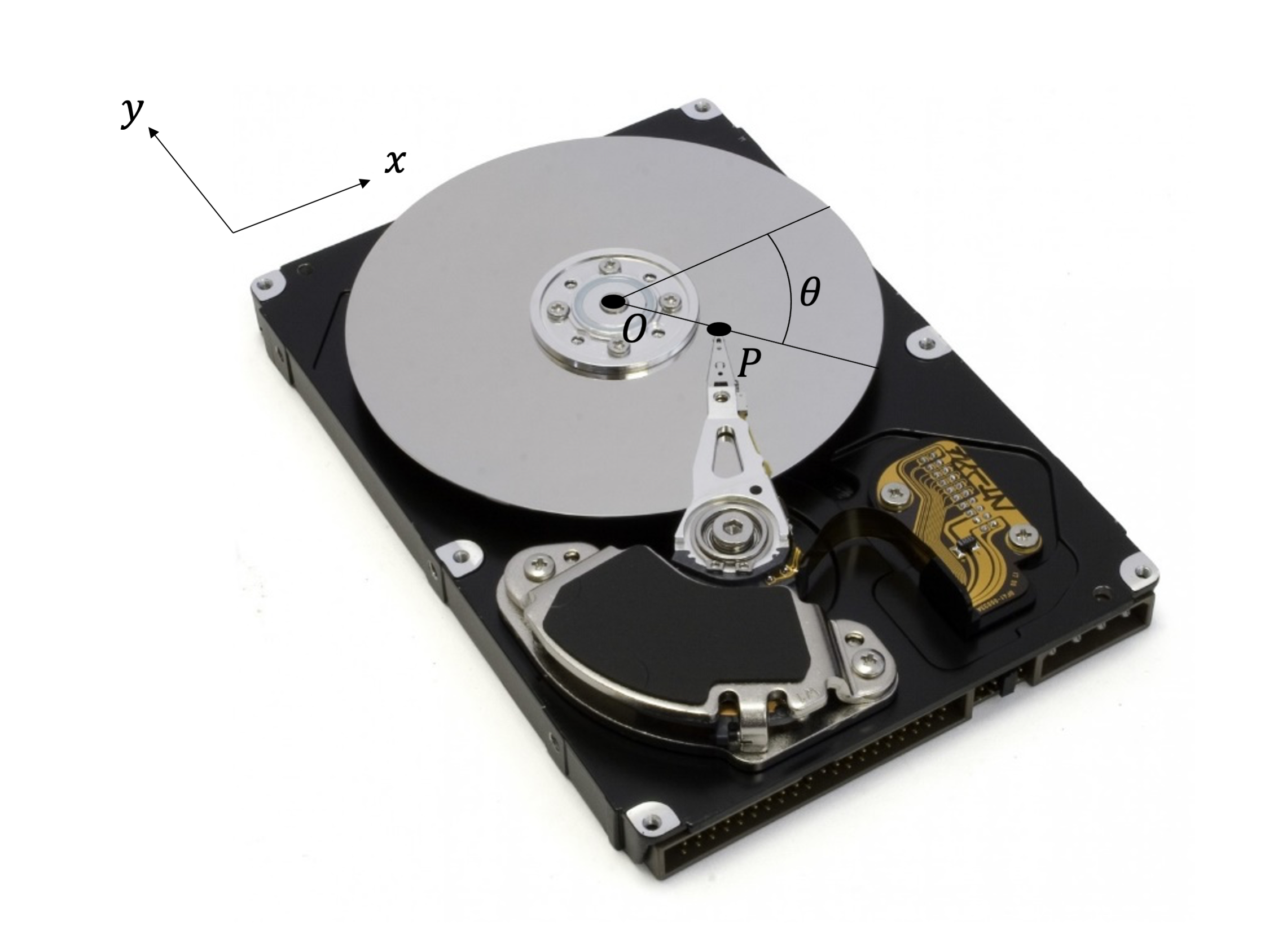### Question 4:

What is the velocity and acceleration of a point, P, on the read/write head of a hard drive? The actuator arm that supports the head has an angular velocity of 40 rad/s clockwise, and an angular acceleration of 200 rad/s2 counter-clockwise. The actuator arm is at 60 degrees above the horizontal at this instant and the distance from P to the arm axle is 3.5 cm.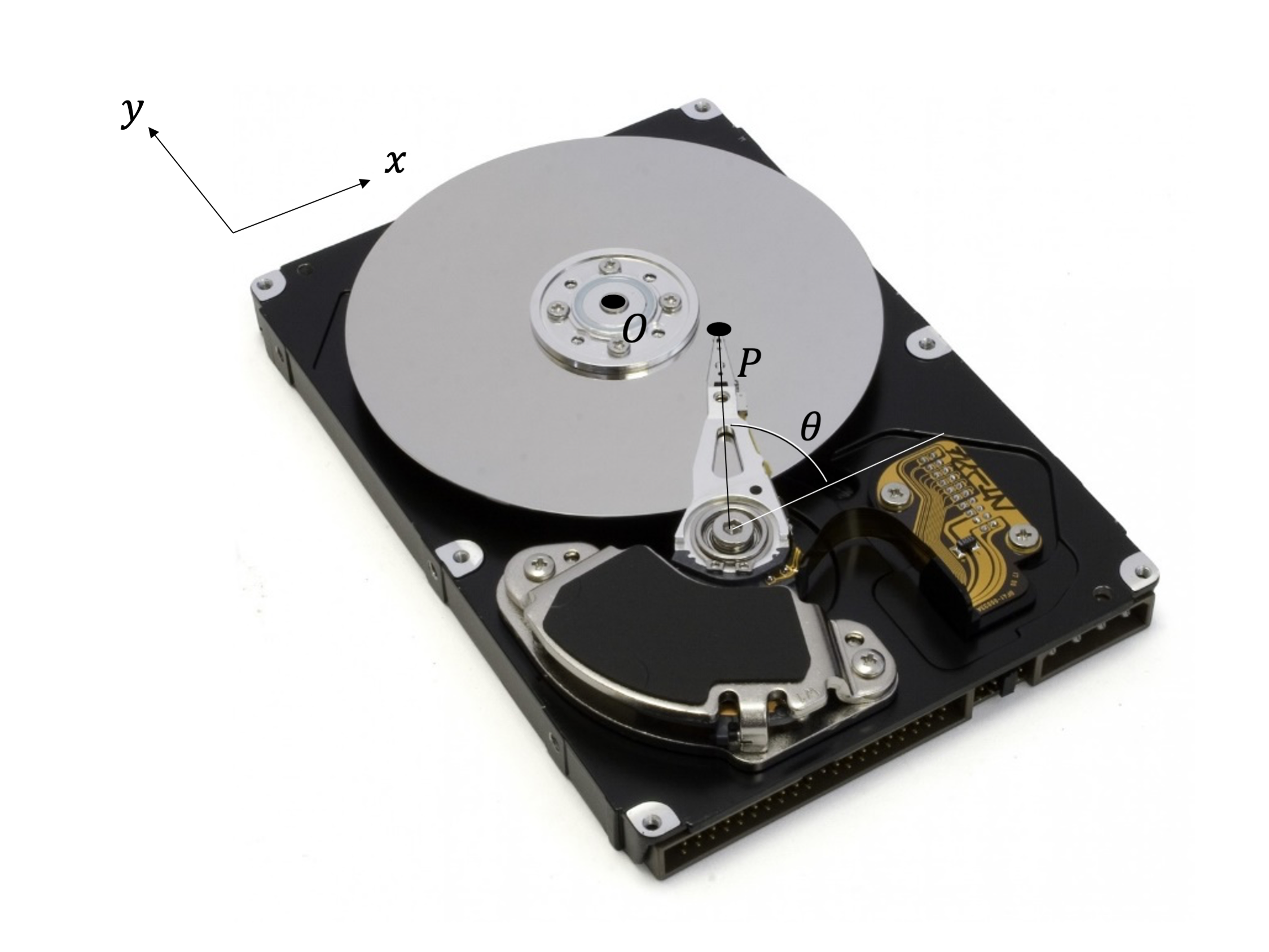### Question 5:

A disk is rotating with an angular velocity ω=2 rad/s and angular acceleration α = 1 rad/s2. Determine the velocity and acceleration of point A, located a distance r = 1m from the center of the disk at a 30 deg angle from the horizontal (θ=30deg).